### Home > PC > Chapter 2 > Lesson 2.3.3 > Problem2-99

2-99.
1. Simplify the following rational expressions. Homework Help ✎

1.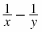2.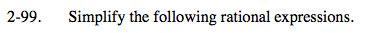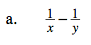Review the Math Notes box.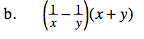$=\left(\frac{y-x}{xy}\right)\frac{(x+y)}{1}$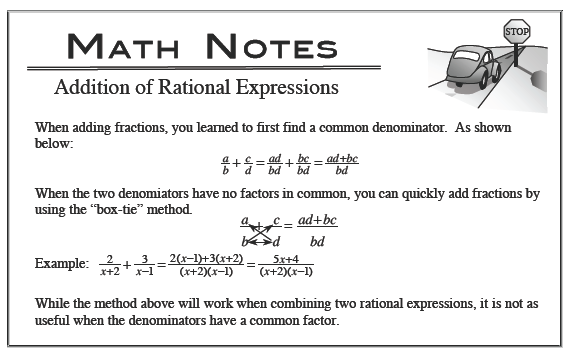$=\frac{xy+y^2-x^2-xy}{xy}=\frac{y^2-x^2}{xy}$